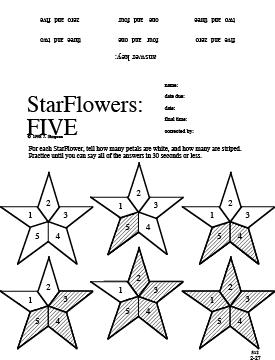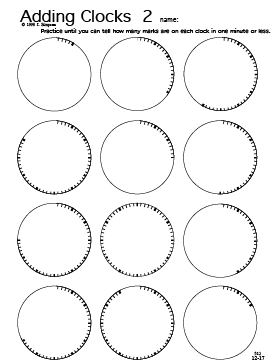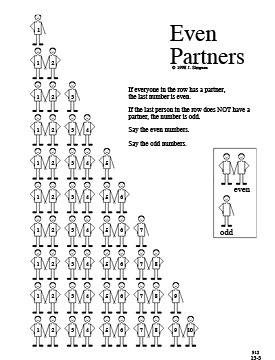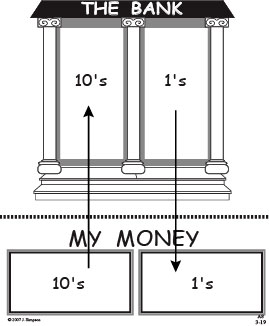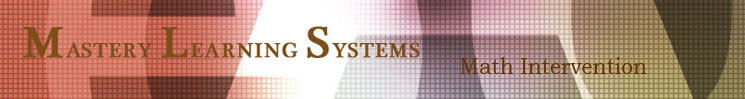K-8 Guided Student Discovery Math for Adding and Subtracting
 Our Books Multiplying and Dividing * The Best Stuff for Multiplying & Dividing * Teams * Discover the Basics - Multiplying & Dividing Adding and Subtracting * Addition, Subtraction, & Place Value * The Best Stuff for Adding & Subtracting * Discover the Basics - Adding & Subtracting Fractions and Decimals * Fractions & Decimals Percents, Ratios, and Rates * Percents, Ratios, & Rates Access to Algebra * Discover the Basics - Equations! * Discover the Basics - Negative Numbers * Discover the Basics - Squares, Roots, & Exponents *Access to Algebra Organizing and Graphing Data * Organizing & Graphing Data Measurement and Geometry * Measurement & Geometry * Discover the Basics - Perimeter, Area, & Volume Math Intervention Strategies

 How it Works Discover and Perfect the power of Guided Student Discovery here: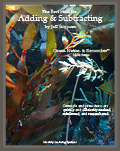512: The Best Stuff for Adding & Subtracting,
420 pages - \$74.95

This is an ideal resource for remedial students, K - 2. There is a lot of material for students to learn to recognize amounts and numerals, and learn to count with a built-in sense of place value. The concepts of addition and subtraction are made abundantly clear with a variety of tactile/visual manipulative strategies. Fluency with the facts is developed from the basis of understanding born of experience. Also addressed is regrouping (borrowing and carrying) missing addends, place value with larger numbers, reading clocks, and even and odd numbers.

 Sample Chapter Headings Plus and Minus 1, Plus and Minus 10 Things That Make Up 10 and 20 Plus and Minus 2, Plus and Minus 20 Regrouping: Carrying Multiple Digits Plus and Minus 8, 80, 800, 8000 ________________________________________________